# 10 Top Tips for GMAT Problem Solving Questions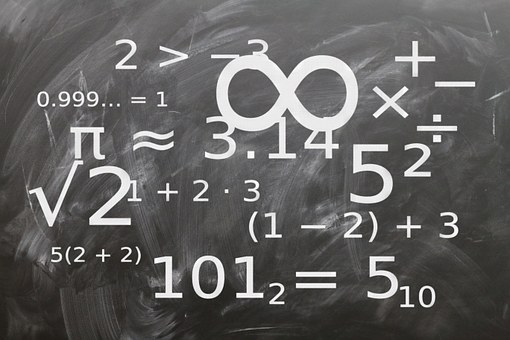For many test takers, the quantitative section of the GMAT is particularly daunting. The challenging section includes two types of questions: data sufficiency and problem solving. While data sufficiency questions are undoubtedly the more notorious question type, GMAT problem solving questions can also be quite tricky.

In this guide, I’ll give you an in-depth look at GMAT problem solving questions. First, I’ll cover what they are and what types of math they cover. Then, I’ll give you the top 10 tips for acing GMAT problem solving questions. Finally, I’ll walk you through solving five sample problem solving questions spanning a variety of topics.

## What Are GMAT Problem Solving Questions?

Problem solving GMAT questions assess how well you can solve numerical problems, interpret graphs and tables, and evaluate information. In plainer language, problem solving GMAT questions are the “traditional” math question type that you’ll see on the GMAT quant section.

While there isn’t a set number of problem solving questions that you’ll see on the GMAT, you can bet that the quant section will be divided just about 50/50 between problem solving and data sufficiency questions. There are 31 total questions on the GMAT quant section, so you there will be either 15 or 16 problem solving questions on the GMAT quant section.

Problem solving questions look a lot like the math questions you’ve seen on other tests. GMAT problem solving questions are all multiple choice questions, with five different answers. Depending on the content tested, problem solving questions may be presented as an equation, a word problem, a diagram, a table, or a graph.

Not sure how or what to study? Confused by how to improve your score in the shortest time possible? We've created the only Online GMAT Prep Program that identifies your strengths and weaknesses, customizes a study plan, coaches you through lessons and quizzes, and adapts your study plan as you improve.

We believe PrepScholar GMAT is the best GMAT prep program available, especially if you find it hard to organize your study schedule and don't want to spend a ton of money on the other companies' one-size-fits-all study plans.Contrary to popular belief, the GMAT quant section doesn’t test on advanced math concepts. The quant section tests your content and analytical knowledge of basic math concepts, such as arithmetic, algebra, and geometry. The same holds true for GMAT problem solving questions – you’ll be asked to apply your knowledge of high school math concepts to questions that are presented in a more challenging and analytical way. For more information about the concepts covered on the GMAT quant section, check out our guide to GMAT quant.

## 10 Tips for Mastering GMAT Problem Solving Questions

Here are the top tips that you can use to master GMAT problem solving questions.

### #1: Master the Fundamentals

GMAT problem solving questions only test high school math concepts. In many ways, this is good news. You’ll have likely encountered every type of math you’ll see on the GMAT before you start studying. Just because the math on the GMAT is relatively basic, however, doesn’t mean that it’s not tricky.

The GMAT tests basic math concepts in complicated ways. Problem solving GMAT questions often ask you to use more than one skill at one time, so you need to have strong mastery of many different concepts.

The key to GMAT problem solving mastery, then, lies in mastering the fundamentals.  Memorize the exponent rules. Memorize common roots and higher powers. Memorize the formulas for finding area of different shapes. Know how to find mean, median, mode, and standard deviation without blinking an eye. Thoroughly understanding the material covered on the GMAT will save you time and boost your score on test day.

### #2: Practice Doing Calculations Without a Calculator

As I mentioned, you won’t be able to use a calculator on the GMAT. As such, you should prepare for problem solving GMAT questions without using a calculator to ensure that you’re used to making basic calculations by hand.

Get used to using scratch paper for calculations and double-checking your work to make sure there are no errors. In particular, make sure that you spend time practicing multiplying and dividing fractions and decimals without a calculator, as you’ll have to do both on the GMAT. The more non-calculator practice you get in before test day, the better prepared and more comfortable you’ll be.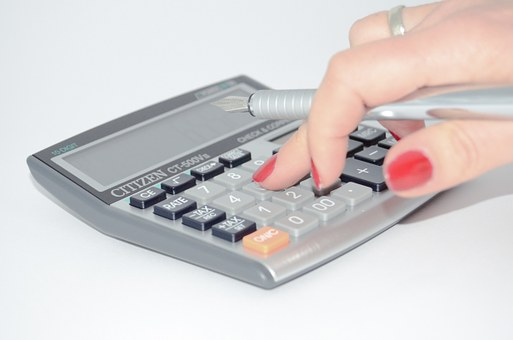You won’t be able to bring a calculator to use on the GMAT, so practice without one!

### #3: Use High-Quality Practice Materials

The best way to prepare for the GMAT is by using real GMAT problem solving questions to practice, since they’re the only questions that simulate the GMAT’s style and content with 100% accuracy. The problem-solving questions have a unique style and logic that many unofficial resources struggle to replicate. Fortunately, there are a ton of real GMAT questions available, and some are even free!

You’ll likely want to supplement each of these resources with other third party tools to help you study. Make sure that any books or online materials you’re using are accurate, useful, and well-respected. A good way to check about the reliability of a book or resource is to read reviews of the resource on Amazon or forums like Beat the GMAT or GMAT Club. We’ve also reviewed the best GMAT books and the best GMAT online resources (coming soon) for you.

### #4: Plug In Numbers

You can solve many GMAT problem solving questions by plugging in real numbers for the variables in equations. Look for questions that have algebraic answers, or questions that ask for the values of algebraic expressions instead of just the values of variables when plugging in numbers. For instance, consider the following question.

If x < y < 0, which of the following is greatest in value?

a. 2x + y
b. x + 2y
c. x – 2y
d. y – 2x

e. 2y – x

For this question, you can pick real numbers that fit the parameters of the question (such as y = -2 and x = -3), and then plug them into each answer to see which answer has the greatest value.

Here are a few tips for plugging in numbers. First, try to use easy, whole integers that fit the constraints of the question. Second, if the question is asking you to determine a value, you can use the numbers yo’ve plugged in to find the matching answer. If the answers are also algebraic terms, keep plugging in your numbers until you get a match. Third, be careful when you’re plugging in numbers! Make sure you go through every one of the answers, as you may find two answers that match with the numbers you’ve chosen. If that’s the case, try plugging in new numbers or solving the problem in a different way, until you’ve only gotten one correct answer.

The writers of the GMAT know that people generally pick positive, whole numbers to plug into their equations. Don’t forget about negative integers, positive and negative fractions, positive and negative decimals, etc., when plugging in numbers to solve a question.

### #5: Work Backwards Using Answer Choices

The GMAT normally arranges answer choices in the ascending numerical value on the quant section. Consider the following example, which we’ll go into more depth on in the next section.

Want to improve your GMAT score by 60 points?

We have the industry's leading GMAT prep program. Built by Harvard, MIT, Stanford, and Wharton alumni and GMAT 99th percentile scorers, the program learns your strengths and weaknesses and customizes a curriculum so you get the most effective prep possible.When Leo imported a certain item, he paid a 7 percent import tax on the portion of the total value of the item in excess of \$1,000 dollars. If the amount of the import tax that Leo paid was \$87.50, what was the total value of the item?

a. \$1,600
b. \$1,850
c. \$2,250
d. \$2,400

e. \$2,750

Notice how the answer choices are written in ascending numerical value. This arrangement means that you can try to plug in an answer and work backwards if you’ve got no idea where to start on a particular question. I’d suggest plugging in the middle answer, so that way you’ll know whether you need to go higher or lower with your answer. You can also use this method to decide which answers to try to plug in next, as well as automatically eliminate the other answers.

### #6: Don’t Rely on Your Eyes

When tackling geometry questions, don’t rely on your eyes to estimate angle sizes, lengths, or areas of figures. Instead, use the numbers provided and your own mastery of geometry concepts. Geometry figures aren’t always drawn to scale, and assuming they are can get you into trouble.

You’ll never encounter a GMAT quant question that you can answer simply by visual estimation. GMAT problem solving questions are designed so that you have to use the information in the question, as well as any information in the diagrams, graphs, charts, or tables, to help you solve the question. That means that you won’t be able to see a triangle and estimate the length of one of its sides just by looking at it. You’ll need to use the information in the question to help you

### #7: Remember That the Numbers Will Work Out

The writers of the GMAT know that you’re not allowed to use a calculator on the quant section. That means that you’ll be able to solve every question using your mastery of fundamental math concepts, a pencil, and scratch paper. If you’re working yourself into a quagmire of exceedingly complicated calculations, stop, take a breath, and reassess the question. You’re likely over-thinking something.

### #8: Use What You Know

No matter how difficult the question may look, remember that you’ll only need to use high school level math to answer it. Start small on questions by using what you know. If you break the problem down to small steps, beginning with what you know, you’ll be able to work towards an answer.

Consider the following sample diagram, which I’ll go into more depth about in the next section.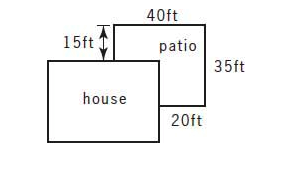Just from looking at this one diagram, I learn about the house and the patio represented. For instance, I know that the western side of the patio is 35 ft long. I know that the eastern side of the patio is 15 ft long. From there, I can determine that the length of the portion of the western wall of the house that intersects with the patio by subtracting 35 – 15.

When you’re approaching GMAT problem solving questions, make sure you’re using all the information in the question and any corresponding charts, tables, or diagrams to find your answer.

One of the keys to success on the GMAT quant section is being able to quickly solve complex math problems. If you can solve most problem solving questions in a minute or less, you’ll have plenty of time leftover to spend on more difficult questions.

To improve your timing, practice with a timer when you’re working on practice sets. Give yourself two minutes to solve every question in your practice set, and see how that feels. Slowly decrease the amount of time you’re giving yourself, until you’re averaging one minute on most questions.

Want to Identify YOUR GMAT Strengths and Weaknesses?

Our proprietary GMAT Diagnostic Assessment creates a customized study plan for you that takes you from registration all the way to test day! It is included with every account and proven to significantly maximize your score.

Get your personalized assessment as part of your 5 day risk-free trial now:As I mentioned in previous tips, you won’t get to use a formula cheat sheet on the GMAT. You’ll have memorize all the formulas you expect to need on test day. You should spend time before test day memorizing the formulas that you’ll see on the GMAT.

Using flashcards is a great way to build your knowledge so that you can quickly recall and use important formulas on test day. Flashcards help you learn to quickly and accurately remember information by forcing you to focus on one small piece of information at a time. Flashcards are also highly portable, and easy to carry with you so that you can practice when you have downtime, such as on your commute to and from work or school. There are many free GMAT flashcard resources out there, but it’s always best to make your own flashcards. In our guide to the best GMAT flashcards, we review the best GMAT flashcard resources out there, as well as tell you the best way to study using flashcards.

## 5 Sample Problem Solving GMAT Questions

These five samples questions will help you see the types of concepts covered in GMAT problem solving questions. Please note: there’s a lot of content covered in GMAT problem solving questions. While I picked sample questions that represent a wide range of topics covered by GMAT problem solving questions, there are many more content areas that you’ll see on the test.

### Problem Solving Sample Question #1

When Leo imported a certain item, he paid a 7 percent import tax on the portion of the total value of the item in excess of \$1,000 dollars. If the amount of the import tax that Leo paid was \$87.50, what was the total value of the item?

a. \$1,600
b. \$1,850
c. \$2,250
d. \$2,400

e. \$2,750

We know that Leo paid \$87.50 of import tax on the total value of an item in excess of \$1,000.

Let’s start by saying that x stands for the total value of the item. We also know that x ≥ 1000, because Leo had to pay import tax on the part that was in excess of \$1,000.

So, (x – 1000) can represent the part that Leo had to pay the 0.7% import tax on.

We can therefore write the equation:

0.7(x – 1000) = 87.50

Multiply both sides by 0.7 to isolate x, which yields us:

X – 1000 = 1250

Add 1000 to both sides to isolate x which yields us:

X = 1250 + 1000

X = 2250.s

The correct answer is C. You could also get the right answer by plugging in the different values. As I mentioned in the tips section, start with the middle number. In this case, plugging in C would yield you the correct answer. However, if it didn’t, you’d be able to use that information to eliminate other answers and decide what to plug in next, as discussed in the earlier tip.### Problem Solving Sample Question #2

If the average (arithmetic mean) of the four numbers 3, 15, 23, and (N+1) is 18, then N =

a. 19
b. 20
c. 21
d. 22

e. 29

This question requires us to understand how to find the arithmetic mean. You find the arithmetic mean of a set of values by dividing the sum of all the values by the total number of values. So, in this case, that yields us the following equation:

3 + 15 + 32 + (n + 1)/4 = 18

3 + 15 + 32 + (n+1) represents the sum of all the values.

4 represents the total number of values.

Now, let’s simplify this equation. In order to isolate n, let’s first multiply each side by 4, which yields us the new equation:

3 + 15 + 32 + (n +1) = 72

We can simplify that equation to get:

51 + N = 72.

Then we can solve for n by subtracting 51 from both sides.

N = 72 – 51

N = 21.

### Problem Solving Sample Question #3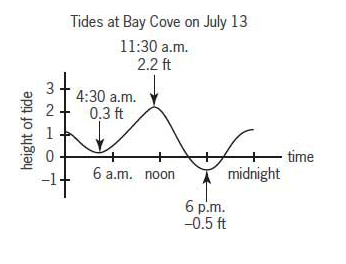The graph above shows the height of the tide, in feet, above or below a baseline. Which of the following is closest to the difference, in feet, between the heights of the highest and lowest tides on July 13 at Bay Cove?

a. 1.7
b. 1.9
c. 2.2
d. 2.5

e. 2.7

This question is all about interpreting graphs. The question asks us to determine the difference between the highest and lowest tides.

First, let’s start off by determining the highest tide. The highest tide seems to be at 11:30 a.m., which is 2.2 ft.

The lowest tide is 0.5 feet below the baseline, which occurs at 6 pm.

Therefore, the equation to express the difference between the heights is [2.2 – (-0.5)] = 2.7 ft.### Problem Solving Sample Question #4A flat patio was built alongside a house as shown in the figure above. If all angles are right angles, what is the area of the patio in square feet?

a. 800
b. 875
c. 1,000
d. 1,100
e. 1,125

You calculate the area of a rectangle by multiplying length x width. 35 x 40 = 1400 ft.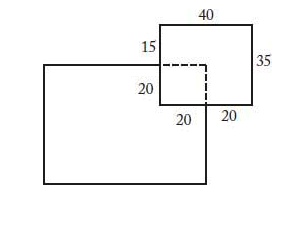Now, because the patio is missing a portion where it intersects with the house, we have to find the area of that missing portion. From the diagram, we can see that the part where the patio intersects with the house is a square with the dimensions 20 ft by 20 ft.

We can find the area of that square by multiplying length times width, so 20 x 20 = 400 ft.

Now to find the area of the patio, we simply subtract 1400 – 400 = 1000 ft.

The patio has an area of 1000 square feet.

### Problem Solving Sample Question #5

Mark and Ann together were allocated n boxes of cookies to sell for a club project. Mark sold 10 boxes less than n and Ann sold 2 boxes less than n. If Mark and Ann have each sold at least one box of cookies, but together they have sold less than n boxes, what is the value of n?

a. 11
b. 12
c. 13
d. 14

e. 15

Let’s start off by defining what we know.

We know that Mark sold 10 less boxes than n. We can express the number of boxes that Mark sold as n – 10.

We know that Ann sold 2 less boxes than n. We can express the number of boxes that Ann sold as n – 2.

We also know that they each sold at least one box of cookies. Thus, we can say that n – 10 ≥ 1 and n – 2 ≥ 1.

Thus, we know that n ≥ 11, because we need at least 11 boxes to make Mark’s statement (n – 10 ≥ 1) true.

We also know that they sold less than n boxes. We can express this as:

(n – 10) + (n – 2) < n. If we solve through for n in this equation, we get that n < 12.

We therefore know that n ≥ 11 and n < 12, which tells us that n = 11.

## Review: How to Attack Problem Solving GMAT Questions

GMAT problem solving questions are more traditional than data sufficiency questions. You’ll see concepts presented in a straightforward way that is very similar to how you’ve seen math questions posed on other standardized tests.

But that doesn’t mean these questions are easy or simple! Problem solving questions cover a wide range of math concepts, from algebra to geometry to number properties and more. Work on mastering fundamental math concepts so that you can work quickly and successfully through problem solving questions on test day.

## What’s Next?

There’s a lot of content covered on the GMAT quant section, so if you’re looking for specific tips on tackling a part content area check out some of our other guides (such as our guides to GMAT percents, probability, and geometry). These guides will help you build up the fundamental knowledge you need to succeed on the GMAT.

Feel like you’ve gotten the hang of GMAT problem solving questions, but wondering what’s up with the other half of the GMAT quant section? Data sufficiency questions are undoubtedly a bit strange, and very different stylistically from any traditional math question you’ve encountered on other standardized tests. Check out our guide to data sufficiency questions to learn more about this unique question type and how to master it.

Wondering how to build in practice on problem solving questions to your GMAT studying? Look no further than our comprehensive GMAT study plan article. In this guide, you’ll find four different GMAT study plans designed to maximize your time and boost your score. You’ll learn how much time you should devote to each section of the test and get recommendations on resources you can use to supplement your practice.